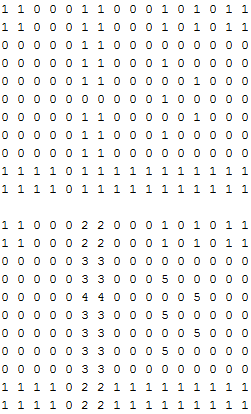## Thursday, April 5, 2012

### Tile Maps: Binary Collision

Back in the day binary collision maps (also known as a type of tile map) were used as a simple and efficient way to check for collision between an object with coordinates of floating point value, and square blocks. What sorts of games used binary collision arrays? Well, pretty much any game that had square tile based levels likely used some form of binary collision, here's a few NES screenshots:ZeldaMarioMetroidZelda
A binary collision array is an array of booleans representing collision or non-collision. In our above examples any square tile that the player could not walk on, or was not empty space would have been represented by a value meaning collision. There would then be a separate array of the same dimensions as the binary collision array, which would represent the different images to draw for each tile.Example Collision and Data Arrays

Above is an example of what the collision and data arrays might look like for the Zelda screenshot with a bridge. Each 1 would be a block that is unwalkable by the character, and each 0 would be a space the character could walk on. The data array holds different numbers, and each number would represent a different image. These image values could perhaps have been stored in some sort of enumeration, or a CBlock in assembly.

Though, how is the character handled? The character in these games seems to have a position of floating point value. I'm not actually sure if they did or didn't for optimization reasons, but what I'll show you uses a floating point coordinate for different characters/enemies. Here's an image showing an example of a floating point coordinate perhaps representing the player's character:

This point would represent the center of the position of the player. The image drawn for this character at this point would be centered directly onto the point. So now how does one go about detecting when the sides of an image on this point are touching a box that represents collision? In order to do that I'll show a method I've learned called "hotspots".

Hotspots are simply points that are offsets of a coordinate, like the coordinate above. The smallest amount of offset hotspots you can have that I'd recommend is four: one for each side of your square image. When implementing a binary collision array for a simple platformer, I found that eight hotspots worked very well. Here's an example image showing eight hotspots on the edges of a square:

Each of those dots are offset by 1/4 of the square's side length, and 1/2 of the square's side length. For example the bottom two hotspots are offset from the center by -1/2 * side length (assuming origin bottom left of the screen). By using these hotspots you can then detect when any of these hotspots points are within the bounds of a binary tile. There are multiple ways to do this, but the way I chose to was to just floor the floating point value of each hotspot and look at only the integer value left. You can do these with an integer typecast, or a floor function. This works since any floating point value in the range of 1.0 through less then 2.0 will still be within the coordinate tile of 1. If this float truncation is confusing, read the next paragraph, if you understand go ahead and skip the next paragraph.

Say you have a coordinate of 3.7 and 4.3 representing one of your hotspots, and you need to check to see if this coordinate is within a tile that represents collision. If you take a look you'll realize that all values from 3.0 to just before 4.0 are all within the x axis on of the value 3 (as in on column 3 of your grid). This goes the same for 4.3; all values from 4.0 through just before 5.0 are in row 4. This means that any coordinate from 3.0 - 3.99999, 4.0 - 4.999999 are within the tile of 3, 4. So then to check to see where any floating point coordinate is on your integer coordinate system, just throw away your decimal value and treat your float as an integer. 3.7 would be 3, and 4.3 would be 4. Thus your resulting coordinate is 3, 4.

Once this is accomplished you can then execute your code to move the character back to the tile they are currently on. This can be done by also flooring the value of the character's coordinate (not the hotspots). However, you want to snap your character back to the closest tile, not just floor the entire floating point value. To do this you use a simple trick:

```coordinate = (int)(coordinate + 0.5f); ```

This will round your floating point number to the nearest tile by offset the decimal portion of your value by 0.5.

Lastly, if you ever implement a coordinate system like here I suggest normalizing your coordinate system. That is have one tile be represented by the value of 1. This allows for simpler mathematics, and allows you to easily transform (scale, rotate, translate) your entire grid/game to whatever exact size you desire. If you normalize your entire grid in your data, you can separate the drawing logic from your data thus decoupling your code overall.

Here's a screenshot for a platformer I worked on for a CS assignment! I had a normalized grid system with the origin represented by the bottom left of the map. I was scaling this map by a factor of 3. I also implemented some simple AI with a statemachine for the red guys! They walk left and right up the edge of their platform, and turn around at the edge, or turn around when they hit a wall.

1.1.